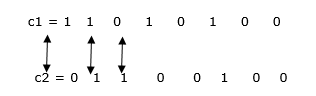# Explain the Hamming Codes in Error Correction

The Hamming code can be used to the data units of any length when we consider the two codewords which have the same length. The Hamming distance between two codewords is represented as the number of positions in which their specific item differs.

ExampleHamming Distance is 3.

Hamming code is also known as linear block code. The family of (n, k) hamming codes for m  is defined.

• Block length n=2m-1.

• The number of message bits k=2m-m-1.

• Number of Parity Bits = n-k=m.

Where m≥3

• Minimum distancemin=3.

• Code rate = Code efficiency = $\frac{k}{n} = \frac{2^{m}-m-1}{2^{m}-1} = 1 - \frac{m}{2^{m}-1}$ of m>> 1

then, code rate r=1 where k is message bits and n is number of transmitted bits per block.

Structure

The parity (redundant) bits are inserted between the data units or the end of data units.

To evaluate, several redundancy bits are needed to accurately represent the given number of data bits m. We should find relationships between m and r.

If a total transmission is m + r, r must indicate m + r + 1 different states. One state defines no error, and m + r states denote the position of an error in each of m + r positions.

Therefore, m + r + 1 states should be discovered by r bits, and r bits can denote 2r multiple states. So 2r ≥m+r+1.

Let us suppose m = 7, then the minimal r-value that satisfies this equation is 4.

 11 10 9 8 7 6 5 4 3 2 1 d D r4 D D D D r3 D r2 r1

In this r bit is put on 2n position. For example, 20 = 1, 21 = 2, 22 = 4 ect positions

In Hamming code, each r bit is VRC (Vertical Redundancy Check).

r1 bit is calculated by using all bit positions whose binary representation contains 1 in the rightmost position, 1, 3, 5, 7, 9, 11.

r2 bit is computed by using all positions with a 1 in 2, 3, 6, 7, 10, 11 positions and so on. r3 bit will take care of bits position at 4, 5, 6. r4 is checked at 8, 9, 10, 11.

Example

1011

11                1001             0111                 0101                  0011               0001

 d D D r4 d d d r3 d r2 r1

r2 will take care of it

1011                1010

11                             10                                 0111     0110                                                             0111     0010

 d D D r6 d d d r4 d r2 r1

and so on.

Calculate r values

We locate each bit of original characters in its suitable position in the 11-bit unit. We can calculate even parities for the multiple bit combinations. The parity value for each consolidation is the value of the correlating r bit.

Example

is calculated to support the even parity for merging bits 3, 5, 7, 9, 11, etc. The last 11-bit code is sent through the transmitted line.